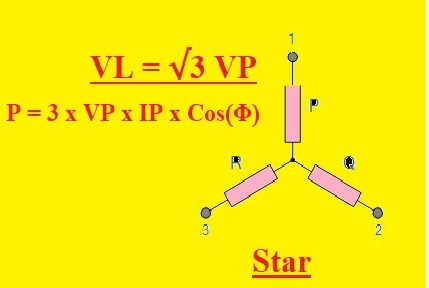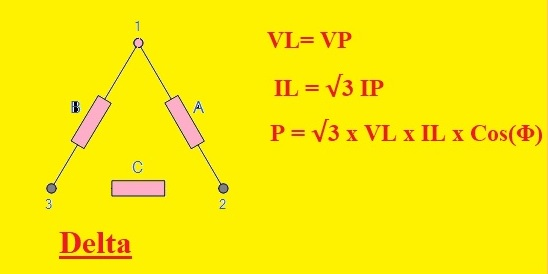Hello, readers welcome to new post. here we will cover the Difference Between Star and Delta Connection. In an electrical power system, there are two main types of connections used for the transmission of power. The first one is star and second one is delta connection each has their own advantages

In this post, we will discuss the basic differences between these two connections. So let get started Difference Between Star and Delta Connection.

## Difference Between Star and Delta Connection

### What is Star Connection

• In the start configuration, all windings have common point at the neutral end. Its structure is like a star and has a neutral wire.
• It helps to protect the overvoltages. In this configuration phase volts is 3 times less than the given volts
• In case of secondary windings can two volts be provided  that are lies among the ends of connections and phase volts among the one phase wire and neutral
• This connection uses less copers which makes it less expensive.
• The configuration of primary and secondary windings to the star in curves is the same to linear current.
• This connection consists of four wires three-phase and one neutral
• It is also called Wye.
• Two main types of star connection four-wire three-phase systems and a three-wire three-phase system
• Its three wires are phases and one is neutral that is a common of three phases
• In this configuration, one point of 3 wires is attached to the mutual to make the Y structure
• Common connection in this structure known as star point or neutral
• Value of phase and line volts are not the same line volts er volts between two phases and phase volts are between phase and neutral.
• Line volts is VL = √3 VP VL is line volts and VP phase volts
• In star configuration, only 2 voltages can be used such as VL and VP. For instance, 230/400 means that VL is four hundred volts and 230 is VP.
• Equation fo power calculation in star connection is P = 3 x VP x IP x Cos(Φ) or P = √3 x VL x IL x Cos(Φ)
• As the value of line volts and phase, volts is not the same so insulation needed for every phase is less in this connection
• This system is used for power transmission and distribution system
• Due to the use of less insulating can be used for long-distance power distribution
• Common applications are where less current at start is needed### What is Delta Connection• Delta is a three-wire connection system
• It has three wire there phase system
• All wires in this system configured in the delta system
• Each wire in its system is connected to a neighboring wire to make a triangle like structure and the common point of these 3 wires make three phases
• This system not have any neutral point
• VL= VP
• It has a single value for votls
• Line current is not the same the phase current  IL = √3 IP
• The equation for measuring the power for this system is P = 3 x VP x IP x Cos(Φ) or P = √3 x VL x IL x Cos(Φ)
• It needed more insulation for phases due to same value of phase and line volts
• Itg used in the distribution systems and for small distances
• It is used for devices where high torque needed

That is all about the Difference Between Star and Delta Connection all details has been explained. If you have any further query ask in comments. Thanks for reading